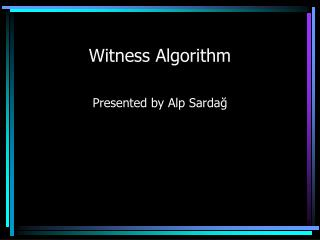DownloadDownload PresentationWitness Algorithm

# Witness Algorithm

Télécharger la présentation## Witness Algorithm

- - - - - - - - - - - - - - - - - - - - - - - - - - - E N D - - - - - - - - - - - - - - - - - - - - - - - - - - -
##### Presentation Transcript

1. Witness Algorithm Presented by Alp Sardağ

2. Witness Algorithm • Like other algorithms, start with a set of vectors, V*t-1={0(t-1),..., M(t-1)}. • Sayfa 81 deki ilk formül • The output of the algorithm will be a set of vectors, V*t-1={0(t-1),..., N(t-1)}.

3. Witness Algorithm • Let Qat the set of vectors representing PLWC value function, for performing action a at time t and performing optimally there after. • Qat = {0a(t),..., Na(t)} • There will be a separate Qat for each action a. • Sayfa 81 10 a kadar olan formüller

4. Witness Algorithm • The algorithm first constructs the Qat sets • Then constructs the desired V*t set of vectors. • In constructing Qat set, the algorithm incrementally build up. • Note that: • Sayfa 81 son formül

5. Constructing Qat • Begin with an empty set Qat, choose any belief point and construct the vector using: • Formula 10 buraya kopyalanacak. • Determine if there exist a belief state * (like Sondik’s): • Sayfa 82 formüllerin ilk iki satırı kopyala. • Once the new vector found, it is added to the list of vectors.

6. Constructing Qat • How the algorithm finds the new belief point? • Sayfa 83 ün sonu ve 84 ün 4 formülü kopyalanacak.

7. Constructing Qat • Sayfa 85 teki LP kopyalanacak.

8. Constructing Qat • The algorithm for constructing Qat is as follows: • Sayfa 85 pseudocode copy paste edilecek.

9. Witness Algorithm Reduction Phase • Once all Qat sets found for each action a, then combine them to form the set V*t that is the optimal value function. • The algorithm uses reduction phase of Monahan’s algorithm. • Monahan’s reduction LP si kopyalanacak.